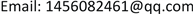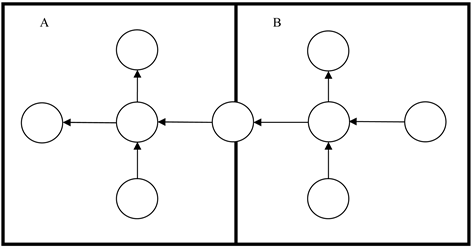﻿ 子网络交叉口信号设计博弈分析 Game Theoretical Intersection Signal Design of Transportation Subnetwork

Operations Research and Fuzziology
Vol.07 No.04(2017), Article ID:22745,4 pages
10.12677/ORF.2017.74015

Game Theoretical Intersection Signal Design of Transportation Subnetwork

Chaowu Tan1, Xiaoning Zhang2

1School of Traffic and Transportation Engineering, Shanghai Maritime University, Shanghai

2School of Economics and Management, Tongji University, ShanghaiReceived: Nov. 3rd, 2017; accepted: Nov. 15th, 2017; published: Nov. 22nd, 2017ABSTRACT

In reality, a transportation network covers different regions. A designer is only responsible for a subnetwork during this region. In this case, signal timing is only for a subnetwork, and managers of different regions compete with each other. In this paper, a subnetwork based signal timing method is established, and the game theoretical behavior among different regions is studied. Applying operational research method, the signal timing problem based on subnetwork is described into a network design problem with user equilibrium constraint, namely the leader-follower game. Numerical example shows that different solutions are obtained when signals are designed based on parallel subnetworks, compared with the integrated signal timing on the whole network.

Keywords:Transportation Network, Signal Timing, Subnetwork, Game Theory

1上海海事大学交通运输学院，上海

2同济大学经济与管理学院，上海1. 引言

2. 子网络交通信号设计问题的描述

${v}_{a}\left(\mu ,x\right)\le {p}_{a}{C}_{a}\left(x\right)\text{,}a\in \stackrel{¯}{A}$ (1)

$k$ 区内，对于进入给定的信号灯交叉口路段 $i\in {I}^{k}$ ，配时变量必须满足一些线性约束，包括信号周期，安全时间，最小和最大绿灯时间等等。这些约束可以用如下的式子表示：

${G}_{i}^{k}{x}_{i}^{k}\ge {b}_{i}^{k}\text{,}i\in {I}^{k}$ (2)

$\underset{{\mu }^{k},\text{}{x}^{k}}{\text{max}}\text{}{\mu }^{k}$ (3)

Subject to

${v}_{a}\left(\mu ,{x}^{k}\right)\le {\mu }^{k}{C}_{a}\left(x\right)\text{,}a\in {\stackrel{¯}{A}}^{k}$ (4)

${G}_{i}^{k}{x}_{i}^{k}\ge {b}_{i}^{k}\text{,}i\in {I}^{k}$ (5)

$\underset{v}{\mathrm{min}}\text{}\underset{a\in A}{\sum }{\int }_{0}^{{v}_{a}}{t}_{a}\left(\omega ,{x}^{k}\right)\text{d}\omega$ (6)

Subject to

$\underset{r\in {R}_{w}}{\sum }{f}_{r}^{w}={\mu }^{k}{q}_{w},\text{}w\in {W}^{k}$ (7)

${v}_{a}=\underset{w\in W}{\sum }\underset{r\in {R}_{w}}{\sum }{f}_{r}^{w}{\delta }_{ar}^{w},\text{}a\in A$ (8)

${f}_{r}^{w}\ge 0,\text{}r\in {R}_{w},\text{}w\in W$ (9)

3. 算例4. 结论

Game Theoretical Intersection Signal Design of Transportation Subnetwork[J]. 运筹与模糊学, 2017, 07(04): 148-151. http://dx.doi.org/10.12677/ORF.2017.74015

1. 1. Abdulaal, M.S. and Leblance, L.J. (1979) Continuous Equilibrium Network Design Models. Transportation Research, 13, 19-32. https://doi.org/10.1016/0191-2615(79)90004-3

2. 2. Wong, S.C. and Yang, H. (1997) Reserve Capacity of a Signal-Controlled Road Network. Transportation Research, 31, 397-402. https://doi.org/10.1016/S0191-2615(97)00002-7

3. 3. Allsop, R.E. (1989) Evolving Applications of Mathematical Optimization in Design and Operation of Individual Signal-Controlled Road Junctions. In: Griffiths, J.D. Ed., Mathematics in Transport Planning and Control, Clarendon Press, Oxford, 1-24.